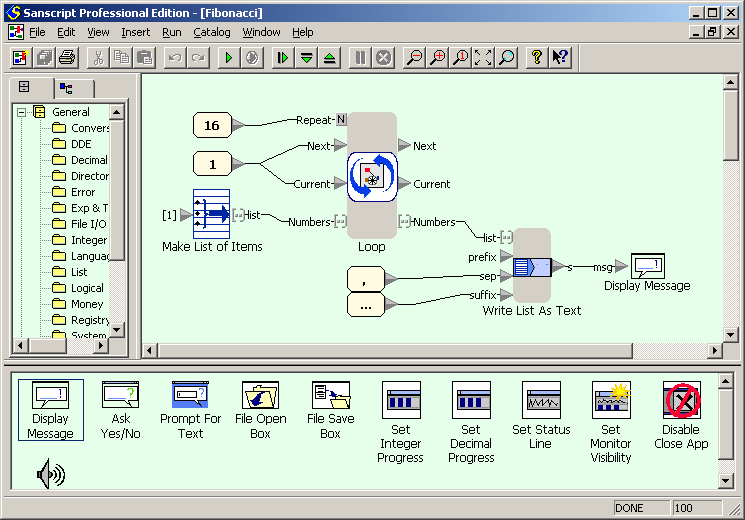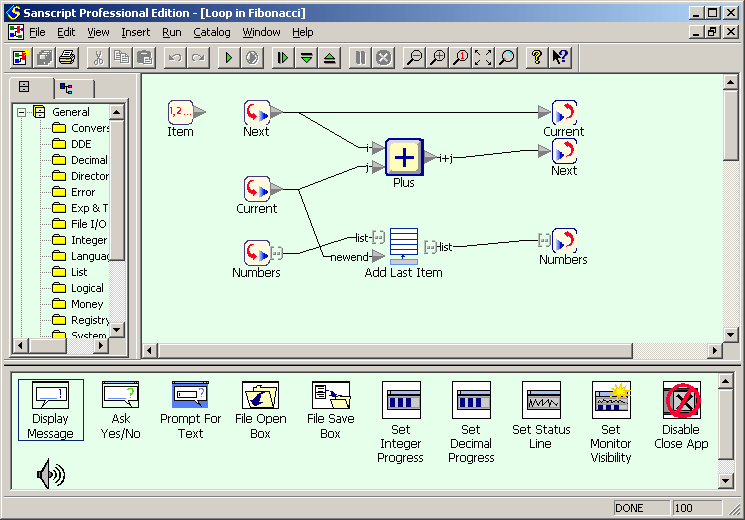Fibonacci numbers in Sanscript

Example for versions Sanscript 2.2

Sanscript is a fully visual programming language, so no source code is available. See screeshots instead.

Fibonacci numbers are calculated in the same way as factorial: a loop calculates a list of numbers, starting with two first ones, and then this list is concatenated to produce the output. Within the loop current number is added to the list and replaced with next number, while next number is replaced with a sum of current and next numbers.Fibonacci numbers example in Sanscript (flowgram)Fibonacci numbers example in Sanscript (repeat)

Top 10 users: